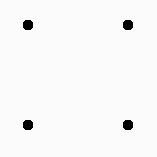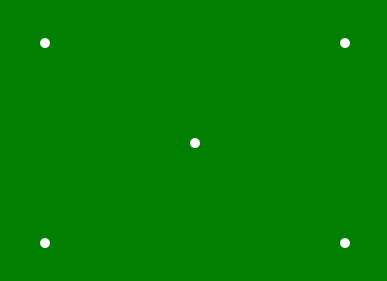Open in App
Not now

# p5.js | point() Function

• Last Updated : 17 Jan, 2020

The point() function is an inbuilt function in p5.js which is used to draw the point in a given coordinate position.

Syntax:

`point( x, y, [z])`

Parameters: This function accept three parameters which are described below

• x: It is used to set the x-coordinate of point.
• y: It is used to set the y-coordinate of point.
• z: It is used to set the z-coordinate in WebGL mode.

Example 1:

 `function` `setup() { ` ` `  `    ``// Create Canvas of given size  ` `    ``createCanvas(400, 300); ` ` `  `} ` ` `  `function` `draw() { ` ` `  `    ``// Use point() function to draw point ` `    ``point(50, 50); ` `    ``point(150, 50); ` `    ``point(50, 150); ` `    ``point(150, 150); ` ` `  `    ``// Use strokeWeight() function to set ` `    ``// the weight of point ` `    ``strokeWeight(10); ` `} `

Output:Example 2:

 `function` `setup() {  ` `     `  `    ``// Create Canvas of given size  ` `    ``createCanvas(400, 300);  ` `}  ` ` `  `function` `draw() {  ` `     `  `    ``// Set the background color ` `    ``background(``'green'``); ` `     `  `    ``// Set the stroke weight ` `    ``strokeWeight(10); ` ` `  `    ``// Set stroke color ` `    ``stroke(``'white'``); ` ` `  `    ``// Draw point ` `    ``point(50, 50); ` `   `  `    ``point(50, 250); ` `   `  `    ``point(350, 50); ` `   `  `    ``point(350, 250); ` `   `  `    ``point(200, 150); ` `   `  `}  `

Output:Online editor: https://editor.p5js.org/
Environment Setup: https://www.geeksforgeeks.org/p5-js-soundfile-object-installation-and-methods/

Reference: https://p5js.org/reference/#/p5/point

My Personal Notes arrow_drop_up
Related Articles